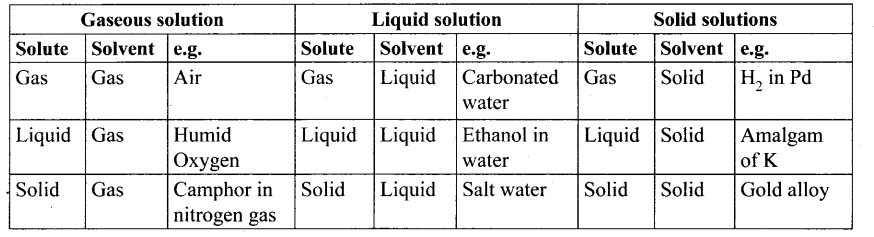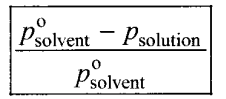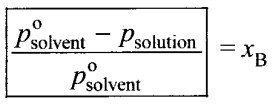## Tamilnadu Samacheer Kalvi 11th Chemistry Notes Chapter 9 Solutions Notes

Solution – It is a homogeneous mixture of two or more substances consisting of atoms, ions or molecules.

Solvent – The compound that is present in large amount in a homogeneous mixture is called solvent.

Solute – The compound that is present in small amount in a homogeneous mixture is called solute.

Aqueous solution – If the water is used as the solvent the resultant solution is called an aqueous solution.

Non-aqueous solution – If solvent other than water is used, then the resultant solution is called non-aqueous solution.

Types and examples of solutionMolality (m) – It is defined as the number of moles of solute present in 1 kg of the solution.

Molarity (M) – It is defined as the number of moles of solute present in 1 L of the solution.

Normality (N) – It is defined as the number of gram equivalents of solute present in 1 L of the solution.

Formality (F) – It is defined as the number of formula weight of the solute present in 1 L of the solution.

Mole fraction – It is defined as the ratio of the number of moles of the component to the total number of moles of all components present in the solution.

Mass percentage – It is the ratio of percentage of the mass of the solute to the mass of solution in grams.

Volume percentage – It is the ratio of percentage of volume of the solute to the volume of the solution in ml.

Mass by volume – It is the ratio of percentage of mass of the solute in g to the volume of the solution in ml.

Parts per million (ppm) – One ppm is equivalent to 1 milligram of the component per litre of solution.

Standard solution – A standard solution is a solution whose concentration is accurately known.

Solubility of a substance – The solubility of a substance is defined as the amount of the solute that can be dissolved in lOOg of the solvent at a given temperature to form a saturated solution.

Factors influencing the solubility – (i) Nature of solute and solvent, effect of temperature, effect of pressure.

Henry’s law – This law states that “the partial pressure of the gas in vapour phase (vapour pressure of the solute) is directly proportional to the mole fraction (x) of the gaseous solute in the solution at low concentration.
PSolute ∝ x solute in solution (or) Psolute = KH .x solute in solution.

KH – ft is the empirical constant with dimensions of pressure.

Evaporation – The process in which the liquid in converted to vapour.

Condensation – The process in which the vapour is converted to liquid.

Vapour pressure – It is defined as the pressure of vapour in equilibrium with its liquid at a given temperature.

Binary solution – The solution which contains only two components is called binary solution.

Raoult’s law – This law states that “in the case of a solution of volatile liquids, the partial pressure of each component (A & B) of the solution is directly proportional to its mole fraction.
PA = K . XA

Dalton’s law of partial pressure – This law states that the total pressure in a closed vessel will be equal to the sum of the partial pressures of the individual components.
pTotal = PA + PB

Ideal solution – It is a solution in which each component i.e., the solute as well as the solvent obeys the Raoult’s law over the entire range of concentration.

Non-ideal solutions – The solutions which do not obey Raoult’s law over the entire range of concentration are called non-ideal solution.

Factors responsible for deviation from Raoult’s law – Solute-solvent interaction, dissociation of solute, association of solute, temperature, pressure, concentration.

Colligative properties – The properties of the solution which do not depend on the chemical nature of the solute particles but depends only on the number of solute particles present in the solution are called colligative properties.

Relative lowering of vapour pressure – It is defined as the ratio of lowering of vapour pressure to vapour pressure of the solvent. Mathematically,Relative lowering of vapour pressure = Moles fraction of solute

Boiling point – It is the temperature at which the vapour pressure of the solution becomes equal to the atmospheric pressure (1 atm).

Elevation of boiling point – ΔTb – Tb – T0b
ΔTb = kb . m where kb = molal boiling point elevation constant.

kb Ebullioscopic constant
$$k_{b}=\frac{\mathrm{RT}_{b}^{2} \mathbf{M}_{\text {solvent }}}{\Delta \mathrm{H}_{\text {vaporisation }}}$$

Freezing point – It is defined as the temperature at which the solid and the liquid states of the substances have the same vapour pressure.

Depression in freezing point ΔTf – ΔTf = kf. m where kf = molal freezing point depression constant.

kf Cryoscopic constant.

Osmosis – It is a spontaneous process by which the solvent molecules pass through a semipermeable membrane from a solution of low concentration to a solution of higher concentration.

Osmotic pressure – The pressure that must be applied to the solution to stop the influx of the solvent (to stop osmosis) through a semipermeable membrane is called osmotic pressure.
π = CRT

Isotonic solutions – Two solutions having same osmotic pressure at a given temperature are called isotonic solutions.

Reverse osmosis (RO) – It can be defined as a process in which a solvent passes through a semipermeable membrane in the opposite direction of osmosis, when subjected to a hydrostatic pressure greater than the osmotic pressure.

van’t Hoff factor (i) – It is defined as the ratio of the actual molar mass to the abnormal molar mass of the solute.

αdissociation = $$\frac{i-1}{n-1}$$

αassociation = $$\frac{(1-i) n}{n-1}$$

Samacheer Kalvi 11th Chemistry Notes